﻿ 结合多通道模板和卷积神经网络的行人检测方法研究 A Study of Pedestrian Detection Based on Multi-Channel Template and Convolution Neural Network

Computer Science and Application
Vol.08 No.01(2018), Article ID:23608,10 pages
10.12677/CSA.2018.81013

A Study of Pedestrian Detection Based on Multi-Channel Template and Convolution Neural Network

Xianyue Pan, Yishan Liu, Ke Tan

Electronic Science and Engineering College, National University of Defense Technology, Changsha Hunan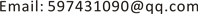Received: Jan. 5th, 2018; accepted: Jan. 23rd, 2018; published: Jan. 30th, 2018ABSTRACT

In this paper, based on the traditional pedestrian detection framework of RGB channel template and convolution neural network, a pedestrian detection method combining multi-channel template and convolution neural network is proposed. The main contribution is: firstly, the motion detection method is used to detect the interest area, improve pedestrian detection efficiency and reduce the false alarm. Secondly, multi-channel template and maximum fusion strategy are adopted to reduce the possible pedestrian leakage detection based on RGB channel template.

Keywords:Channel Template, Convolutional Neural Network, Pedestrian Detection1. 引言

2. 感兴趣区域提取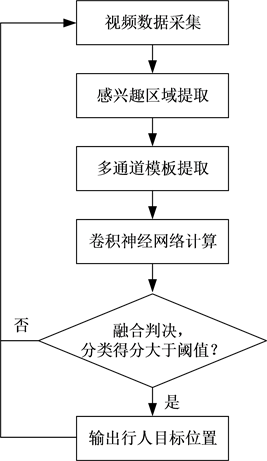Figure 1. The pedestrian detection process based on multi-channel templates and CNN

$P\left({X}_{t}\right)=\sum _{k=0}^{K}{\omega }_{k,t}{\eta }_{k}\left({X}_{t},{\mu }_{k,t},{\Sigma }_{k,t}\right)$ (1)

${\eta }_{k}\left({X}_{t},{\mu }_{k,t},{\Sigma }_{k,t}\right)=\frac{1}{\sqrt{{\left(2\text{π}\right)}^{n}|{\Sigma }_{k,t}|}}\mathrm{exp}\left(-\frac{1}{2}{\left({X}_{t}-{\mu }_{k,t}\right)}^{\text{T}}{\Sigma }_{k,t}{}^{-1}\left({X}_{t}-{\mu }_{k,t}\right)\right)$ (2)

$B=\underset{b}{\mathrm{arg}\mathrm{min}}\left(\sum _{k=1}^{b}{\omega }_{k,t}>{T}_{W}\right),\text{\hspace{0.17em}}b=1,2,\cdots ,K$ (3)

${\omega }_{k,t}=\left(1-\alpha \right){\omega }_{k,t}+\alpha {M}_{k,t}$ (4)

$|{X}_{t}-{\mu }_{k,t-1}|\le 2.5{\sigma }_{k,t-1}$ (5)

$\left\{\begin{array}{l}{\mu }_{k,t}=\left(1-\rho \right){\mu }_{k,t-1}+\rho {X}_{t}\\ {\sigma }_{k,t}^{2}=\left(1-\rho \right){\sigma }_{k,t-1}^{2}+\rho {\left({X}_{t}-{\mu }_{k,t-1}\right)}^{2}\end{array}$ (6)

$\rho =\alpha {\eta }_{k}\left({X}_{t}|{\mu }_{k,t-1},{\Sigma }_{k,t-1}\right)$ (7)

3. 多通道模板提取

${f}_{RGB}^{\left(R\right)}\left(x,y\right)$${f}_{RGB}^{\left(G\right)}\left(x,y\right)$${f}_{RGB}^{\left(B\right)}\left(x,y\right)$ 分别表示像素点 $\left(x,y\right)$ 在RGB_CM上的R通道值、G通道值和B通道值， ${f}_{Y}\left(x,y\right)$ 表示像素点 $\left(x,y\right)$ 在Y_CM上的像素值， ${f}_{LBP}\left(x,y\right)$ 表示像素点 $\left(x,y\right)$ 在LBP_CM上的像素值， ${f}_{G}\left(x,y\right)$ 表示像素点 $\left(x,y\right)$ 在G_CM上的像素值，那么有

${f}_{Y}\left(x,y\right)=0.3{f}_{RGB}^{\left(R\right)}\left(x,y\right)+0.59{f}_{RGB}^{\left(G\right)}\left(x,y\right)+0.11{f}_{RGB}^{\left(B\right)}\left(x,y\right)$ (8)Figure 2. Schematic diagram of four channel templates

${f}_{G}\left(x,y\right)=\sqrt{{\left({f}_{Y}\left(x+1,y\right)-{f}_{Y}\left(x-1,y\right)\right)}^{2}+{\left({f}_{Y}\left(x,y+1\right)-{f}_{Y}\left(x,y-1\right)\right)}^{2}}$ (9)

${f}_{LBP}\left(x,y\right)=\sum _{n=1}^{8}{f}_{LBP}^{\left(n\right)}\left(x,y\right){2}^{n-1}$ (10)

${f}_{LBP}^{\left(n\right)}\left(x,y\right)=\left\{\begin{array}{l}1,\text{\hspace{0.17em}}\text{\hspace{0.17em}}\text{\hspace{0.17em}}\text{\hspace{0.17em}}\text{\hspace{0.17em}}{f}_{Y}^{\left(n\right)}\left(x,y\right)\ge {f}_{Y}\left(x,y\right)\\ 0,\text{\hspace{0.17em}}\text{\hspace{0.17em}}\text{\hspace{0.17em}}\text{\hspace{0.17em}}\text{\hspace{0.17em}}其他\end{array}$ (11)

4. 卷积神经网络计算

${x}^{l}={f}^{l}\left({x}^{l-1},{\theta }^{l}\right)$ (12)

${\theta }^{l}=\left[{w}^{l},{b}^{l}\right]$ (13)

${f}^{l}$ 表示第l层的网络模型，也即该层网络所要执行的操作，主要分为四种类型：卷积、池化、全连接和回归。

CNN计算将输入的每一个图像映射到每一个类别的分类得分可以表示为

${p}_{c}=\text{softmax}\left({x}^{L-1}|{\theta }^{L}\right)$ (14)

$\text{softmax}\left({x}^{L-1}\right)=\frac{\mathrm{exp}\left({x}_{c}^{L-1}\right)}{\mathrm{exp}\left({x}_{0}^{L-1}\right)+\mathrm{exp}\left({x}_{1}^{L-1}\right)}$ (15)

${x}_{{m}^{\prime },{n}^{\prime }}^{l}=\sum _{m}\sum _{n}\left({w}_{m,n,{d}^{\prime }}^{l}{x}_{m+{m}^{\prime },n+{n}^{\prime }}^{l-1}+{b}_{{d}^{\prime }}^{l}\right)$ (16)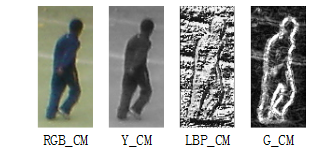Figure 3. Neighborhood pixel sequence number schematic

$a\left({x}_{{m}^{\prime },{n}^{\prime }}^{l}\right)=\mathrm{max}\left(0,{x}_{{m}^{\prime },{n}^{\prime }}^{l}\right)$ (17)

${x}_{m,n}^{l}=\underset{{m}^{\prime },{n}^{\prime }\in L\left(m,n\right)}{\mathrm{max}}\left({x}_{{m}^{\prime },{n}^{\prime }}^{l-1}\right)$ (18)

5. 融合判决

${p}_{1}=\mathrm{max}\left({p}_{1}^{\left(RGB_CM\right)},{p}_{1}^{\left(\text{Y}_CM\right)},{p}_{1}^{\left(\text{LBP}_CM\right)},{p}_{1}^{\left(\text{G}_CM\right)}\right)$ (19)

6. 实验与分析

6.1. 行人检测数据集

1) INRIA

INRIA是最早使用的行人检测数据集，该数据集包含的图像共有5264幅(其中包含行人的图像有3548幅)。尽管该数据集规模不大，但优势在于包含的行人图像是经过裁剪的，非常适用于行人检测器的训练。

2) Caltech

Caltech数据集是由穿越美国洛杉矶街头的车辆记录的2.5小时30 Hz视频构成的，视频分辨率为640 × 480。视频中注释了共计35万个包围约2300个独立行人的包围盒，也即人工标注的行人窗口数量约为350,000个。由于这些数据都是来自真实街区场景的自然行人，行人存在遮挡现象和姿态变化，因此检测难度较大。

6.2. 常用性能评价指标

$\text{TPR}=\frac{正确检测到的行人数量}{标记的行人数量}×100%$ (20)

$\text{FPR}=\frac{错误检测到的行人数量}{错误检测到的行人数量+正确检测到的行人数量}×100%$ (21)

${\text{IoU}}^{\left(r,\stackrel{˜}{r}\right)}=\frac{{\stackrel{⌢}{S}}^{\left(r,\stackrel{˜}{r}\right)}}{{S}^{\left(r\right)}+{S}^{\left(\stackrel{˜}{r}\right)}-{\stackrel{⌢}{S}}^{\left(r,\stackrel{˜}{r}\right)}}×100%$ (22)

6.3. 不同方法的性能对比分析

Neural Networks)进行行人目标检测，简记为DCNN方法。文献  是一种结合运动检测和特征分类的行人检测方法，采用光流法检测运动区域，然后结合HOG特征和SVM分类器进行行人的检测，简记为OF_HOG_SVM方法。这三种方法可以从不同的侧面来印证本文方法的优越性。需要说明的是，四种方法都使用INRIA数据集、Caltech数据集的set00~set05子集以及自建数据集的前20个视频片段进行训练。测试时，首先在Caltech数据集的set06~set10子集上进行测试，然后再在剩余的自建数据集上进行测试，分别统计各个数据集上的行人检测性能指标。其中，图4展示了Caltech数据集上四种方法的性能指标。图5展示了自建数据集上四种方法的性能指标。表2给出了四种方法的平均检测耗时对比结果。下面结合实验结果进行分析。

1) FPR指标对比分析

2) TPR指标对比分析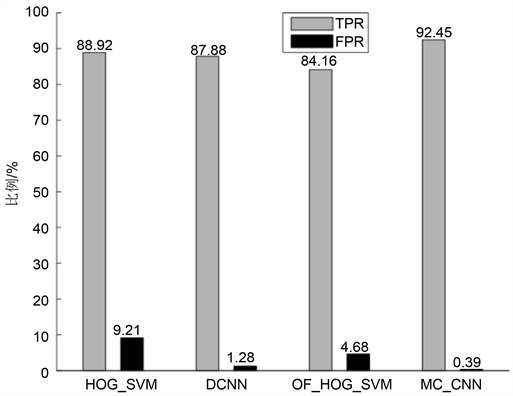Figure 4. Caltech data set test results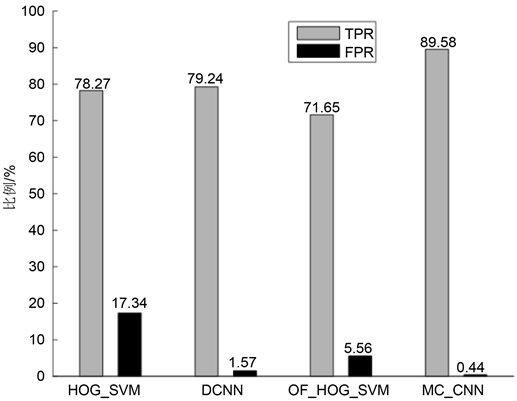Figure 5. Self-built data set test results

3) 运算效率对比分析Table 2. Average time-consuming comparison of four methods under two data sets

7. 结束语

A Study of Pedestrian Detection Based on Multi-Channel Template and Convolution Neural Network[J]. 计算机科学与应用, 2018, 08(01): 97-106. http://dx.doi.org/10.12677/CSA.2018.81013

1. 1. 李权. 面向安全监控的异常声音识别的研究[D]: [硕士学位论文]. 长沙: 湖南师范大学, 2015.

2. 2. 张永良, 张智勤, 吴鸿韬, 等. 基于改进卷积神经网络的周界入侵检测方法[J]. 计算机科学, 2017, 44(3): 182- 186.

3. 3. Ribeiro, D., Nascimento, J.C., Bernardino, A., et al. (2016) Improving the Performance of Pedestrian Detectors Using Convolutional Learning. Pattern Recognition, 61, 611-619.

4. 4. Zhou, Z. and Xu, L. (2014) Pedestrian Detection Based on HOG and Weak-Label Structural SVM. Journal of Computational Information Systems, 10, 367-374.

5. 5. Tomè, D., Monti, F., Baroffio, L., et al. (2016) Deep Convolutional Neural Networks for Pedestrian Detection. Image Communication, 47, 482-489. https://doi.org/10.1016/j.image.2016.05.007

6. 6. Zhang, S., Bauckhage, C., Klein, D.A., et al. (2016) Moving Pedestrian Detection Based on Motion Segmentation. Multimedia Tools & Applications, 75, 6263-6282. https://doi.org/10.1007/s11042-015-2571-z